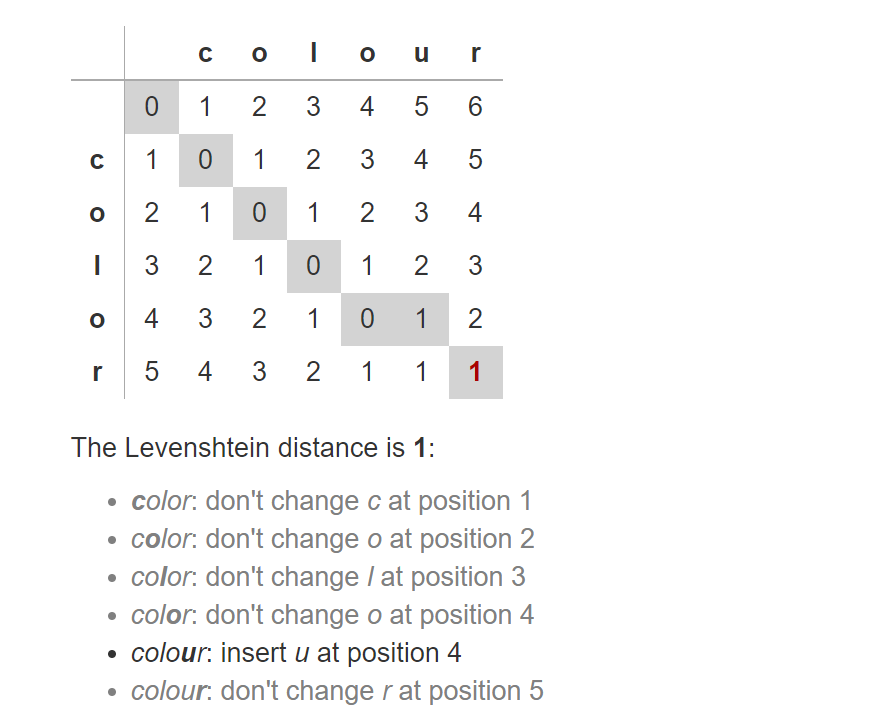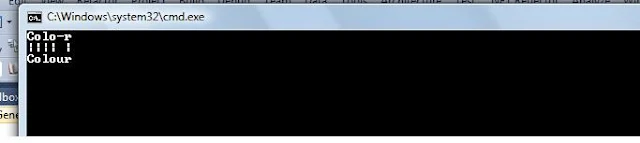# Levenshtein distance (Edit distance) - C#

Learn how to implement the Levenshtein distance algorithm in C# to measure the number of changes required to transform one string into another. Explore the step-by-step implementation and see how to print the result. Get insights into the concept and usage of the Levenshtein distance.

## Introduction:

In this article, we will dive into the implementation of the Levenshtein distance algorithm in C#. The Levenshtein distance is a metric used to measure the number of edits (insertions, deletions, or substitutions) required to transform one string into another. It is named after Soviet mathematician Vladimir Levenshtein, who introduced it in 1966. By understanding and implementing this algorithm, you can gain insights into measuring string similarity and solving various text-related problems.

1. What is the Levenshtein Distance Algorithm?
2. Implementation of the Levenshtein Distance Algorithm in C#
3. Example: Finding the Edit Distance between “color” and “colour”
4. Conclusion

## 1. What is the Levenshtein Distance Algorithm?

The Levenshtein distance algorithm calculates the minimum number of edits needed to transform one string into another. These edits can involve replacing a letter, inserting a letter, or deleting a letter. The algorithm considers the costs associated with each edit and finds the optimal combination of edits that results in the minimum overall cost. The Levenshtein distance is widely used in fields like computational linguistics, spell checking, and DNA sequence analysis.

The edit distance of two strings, s1 and s2, is defined as the minimum number of point mutations required to change s1 into s2, where a point mutation is one of:

1. Replace a letter,
2. Insert a letter, or
3. Delete a letter

Let’s consider the two words `color` and `colour` if you apply the above logic you will find that edit distance is 1 because you have to insert one character `u` to make color to colour.## 2. Implementation of the Levenshtein Distance Algorithm in C#

To implement the Levenshtein distance algorithm in C#, we follow these steps:

• Create a character map to store the costs of all edits.
• Initialize the character map by assigning initial values for insertion and deletion operations.
• Iterate through the strings and compare characters to calculate the costs of each edit operation.
• Update the character map by selecting the minimum cost among deletion, insertion, and substitution.
• Print the path of edits that lead to the minimum edit distance.
• Finally, return the minimum edit distance between the two strings.

## 3. Example: Finding the Edit Distance between “color” and “colour”

Let’s consider an example to calculate the edit distance between the words “color” and “colour.” By applying the Levenshtein distance algorithm, we find that the edit distance is 1. We need to insert the character ‘u’ to transform “color” into “colour.”

Below is the example code that demonstrates the Levenshtein distance algorithm in C#:``````void Main()
{
EditDistanceAlgo.LevenshteinDistance("color", "colour");
}

public class EditDistanceAlgo
{
private static int[,] m;
private static char diagonalCharacter;
public static int LevenshteinDistance(string first, string second)
{
int[,] characterMap = new int[first.Length + 1, second.Length + 1];
for (int i = 0; i <= first.Length; i++)
characterMap[i, 0] = i;
for (int j = 0; j <= second.Length; j++)
characterMap[0, j] = j;
for (int j = 1; j <= second.Length; j++)
for (int i = 1; i <= first.Length; i++)
if (first[i - 1] == second[j - 1])
characterMap[i, j] = characterMap[i - 1, j - 1];  //no operation
else
characterMap[i, j] = Math.Min(Math.Min(
characterMap[i - 1, j] + 1,    //a deletion
characterMap[i, j - 1] + 1),   //an insertion
characterMap[i - 1, j - 1] + 1 //a substitution
);
PrintPath("", "", "", characterMap, first.Length, second.Length, first, second);
return characterMap[first.Length, second.Length];
}
private static void PrintPath(string row1, string row2, string row3, int[,] characterMap, int i, int j, string first, string second)
{
string result = "";
if (i > 0 && j > 0)
{
var diag = characterMap[i - 1, j - 1];
diagonalCharacter = '|';
if (first[i - 1] != second[j - 1])
{
diag++; diagonalCharacter = '\t';
}
if (characterMap[i, j] == diag)
PrintPath(first[i - 1] + row1, diagonalCharacter + row2, second[j - 1] + row3, characterMap, i - 1, j - 1, first, second);    // change or match
else if (characterMap[i, j] == characterMap[i - 1, j] - 0 + 1) // delete
PrintPath(first[i - 1] + row1, ' ' + row2, '-' + row3, characterMap, i - 1, j, first, second);
else
PrintPath('-' + row1, ' ' + row2, second[j - 1] + row3, characterMap, i, j - 1, first, second);      // insertion
}
else if (i > 0)
PrintPath(first[i - 1] + row1, ' ' + row2, '-' + row3, characterMap, i - 1, j, first, second);
else if (j > 0)
PrintPath('-' + row1, ' ' + row2, second[j - 1] + row3, characterMap, i, j - 1, first, second);
else // i==0 and j==0
result += row1 + '\n' + row2 + '\n' + row3 + '\n';
Console.WriteLine(result);
}
}
``````

## Conclusion

In this tutorial, we learned how to implement the Levenshtein distance algorithm in C#. By understanding the steps involved and analyzing the example code, you can apply this algorithm to measure the edit distance between two strings. The Levenshtein distance has various applications, such as spell checking, text analysis, and DNA sequence alignment. Feel free to experiment with different strings and explore further modifications of the algorithm to suit your specific needs.

Remember to practice implementing and using the Levenshtein distance algorithm to enhance your problem-solving skills and expand your knowledge in the field of string manipulation. Happy coding! 😊

#### Related article

How to Implement a "Did you mean by " in JavaScript